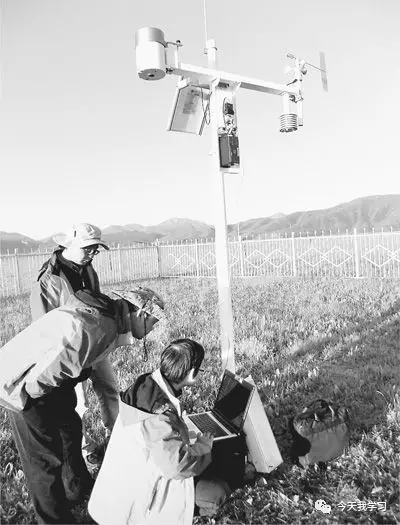## var thirdLink = \$(".current-center").children("a.CurrChnlCls"); var thirdText = \$(thirdLink).text(); var now; if(thirdText == "高层声音"){ now = ''; now += " ："; document.write(now); }else if((thirdText == "专家视点") || (thirdText == "领导心得")){ now = ''; now += " ："; document.write(now); } 一群扎根荒原“掘金”的科研“追梦人”